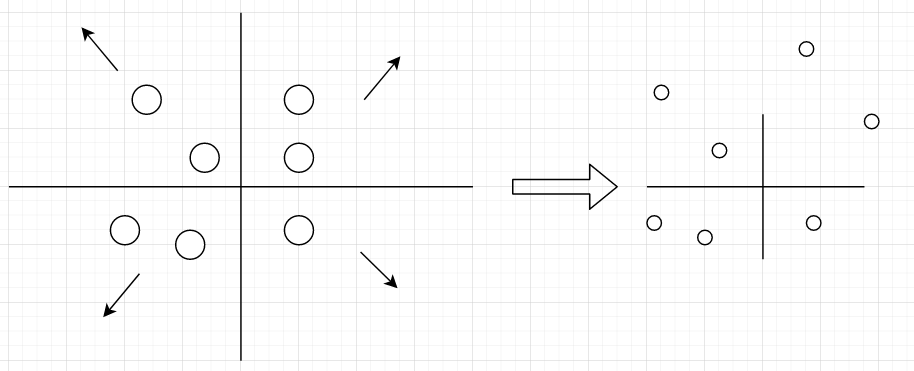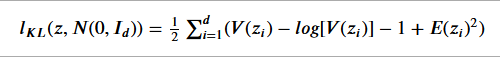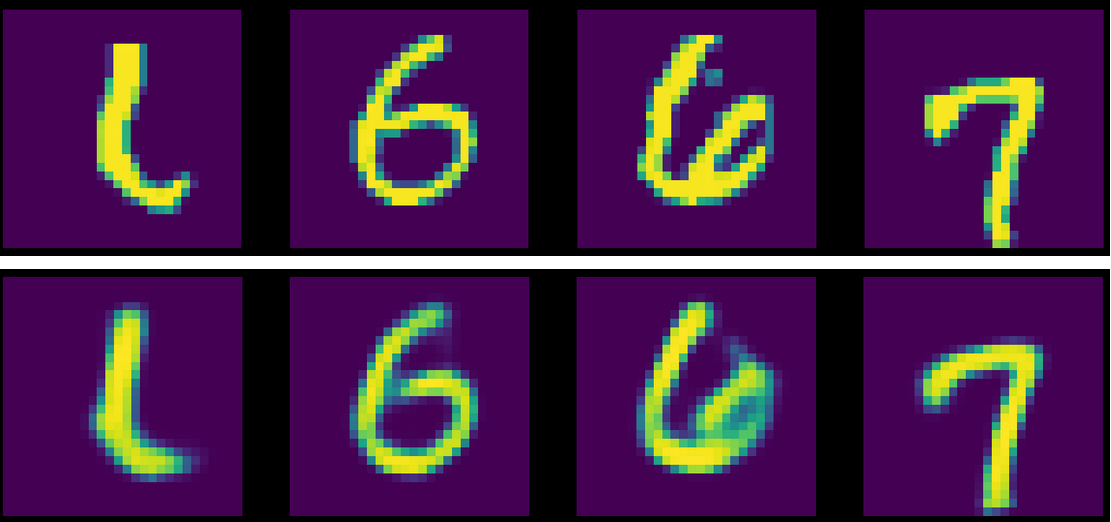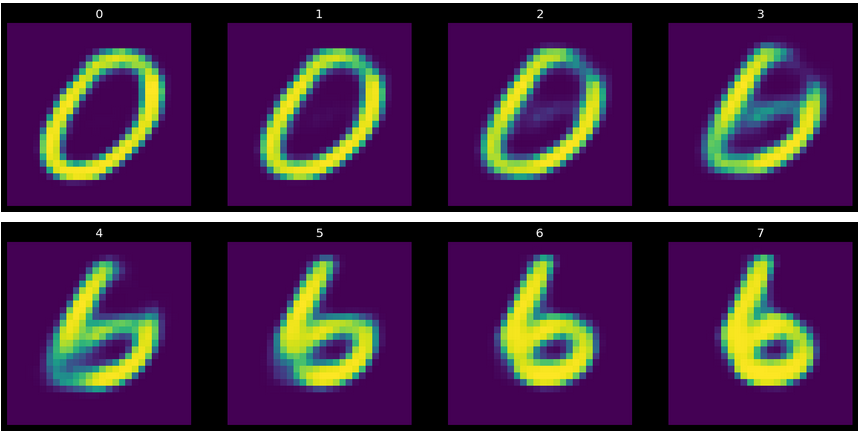# Introduction

In previous posts on autoencoders (Part 1 & Part 2), we explored the intuition, theory and implementation of under and over-autoencoders. The autoencodes have two parts: encoder and decoder. The encoder moves the input to the latent space, while the decoder tries to get the input representation back from the latent space representation.

## The problem with classical autoencoders

However, the encoder in these cases are deterministic in nature, i.e. the map a value in input to a point in latent space, which is subsequently mapped back to input space by decoder. However, a good autoencoder should not be learning point representation of input data, but more of a distribution of the latent space features owing to two reasons:

• The characteristics of data do not have a point representation. They have a distribution.
• The manifold structure of the input data should be smooth and not disconnected.

Varational autoencoders(VAE) try to address these issue by using a probabilistic model of latent representations which understands the underlying causal relations better, helping in more effective generalization.

# Structure

Consider z, the latent space or hidden representation, and input x, and let z have a probability distribution p(z).

For generalization, we would like to have p(x). We have access to only x, we would like to capture p(z|x).

So, to capture p(x), we need p(z). However, as z is inaccessible, we cannot know the distribution of z and subsequently p(z), making this problem intractable.

However, there is another way to solve this issue. Latent variable z can be forced to follow a known distribution. This what VAE does. In VAE, we enforce Gaussian distribution on latent variable z. Gaussian distribution can be characterized by the mean and the variance, which are estimated by the input values.

VAE has 3 components:

• Encoder: It encodes the input vector in n dimensions into 2h dimensions. h is the dimensions of the latent space. Here, 2h dimensions represent the h means and h variances concatenated.
• The sampler: It takes the 2h dimensional long vector and creates Gaussian distribution based on the h means and h variances, from which the z value used for output is sampled.
• The decoder: It takes the Gaussian distribution and outputs the reconstructed vector, which is compared to the input vector to calculate the loss.

## How to enforce structure in latent variables distribution

Let’s consider what the classical autoencoder loss function, i.e reconstruction loss does here. The distribution of z can be thought as bubbles in latent space. The reconstruction loss is minimized when the bubbles don’t overlap at all, as the overlap will bring a point of ambiguity. Hence, the reconstruction loss pushes the bubbles of z distribution as far as possible from each other. If there is no regulation on how far these bubbles can lie, the reconstruction loss would would push them too far apart, making the distribution negligible compared to the space in which bubbles are located, defeating the whole purpose of the getting the distribution in the first place.z distribution bubbles floating away thanks to reconstruction loss. Source: author

Hence, we need a term in loss which can tie the distribution bubbles down, and enforce the Gaussian distribution on individual bubbles. This role is done by KL divergence of the Gaussian distribution with respect to the normal distribution with zero mean and unit variance.

The KL divergence(or relative entropy) of the Gaussian with respect to a standard normal distribution is:KL divergence of Gaussian distribution with mean E(z) and variance V(z). source: author

The last term of the KL divergence puts a L2 penalty on the means of the latent variables, pulling them towards the origin, stopping them from wandering away. The rest of terms i.e. `V(z)-log(V(z))-1` has a minima at V(z) at 1 which is obvious at we are finding the relative entropy with respect to the standard normal distribution. Hence, the composite loss term strikes a balance between reduction of reconstruction error and the difference from the standard normal distribution.

## The reparameterisation trick

We do the sampling from the Gaussian distribution for the latent variable (z) which is fed to the decoder. However, it creates a problem in backpropagation and consequently optimizing, because when we do gradient descent to train the VAE model, we don’t know how to do backpropagation through the sampling module.

Instead, we use reparameterisation trick to sample for z.

Now, the backpropagation is possible, as the gradients with respect to z have to go through the sum and product functions only.

# Implementation using PyTorch

We will be using the MNIST dataset for explanation.

The data loading and transformation steps are similar to the classical encoders. We will be focusing on the architecture of the VAE here.

We define a simple VAE in terms of 3 units, encoder, decoder and sampler. The sampler implements the reparameterisation trick discussed above.

## Base architecture

The input dimensions(784) correspond to the MNIST image size of 28x28. The input data is moved from 784 dimensions to 400(dxd) dim, passed through non-linear layer (ReLU) and then moved to 2d hyperspace, where d = 20. The latent space is d(20) dimensional. We need 2d dimensions as it is concatenation of d means and d variances.

The decoder module is also very similar to classical autoencoder. It takes a d dimensional latent space vector, passes it through set of linear and non-linear layers and outputs final vector of size 784, same as input.

The magic happens in the sampler layer.

The sampler module takes the 2d vector and returns z based on reparameterisation trick. One thing to note here is that we do not input variance into the sampler layer, but log of variance. It is so as variance has to be positive, while log of variance can be negative too. It makes sure that the variance is always positive and we are able to use the full range of values as input. It makes the process more stable too.

Next we define the loss as sum of reconstruction loss and KL divergence. The training is similar to classical autoencoder and has been covered in earlier article.

Before training, this is the reconstruction we are getting, where top row is the original, and bottom is reconstruction:

After just 20 epochs, following are the results of the reconstruction:Actual vs reconstruction by VAE after training. source: author

To look at the latent space vectors, we generate few random samples and use decoder to generate a sample:

`z_sample = torch.randn((8, d)).to(device)sample_out = model.decoder(z_sample)`

We can see that the latent space has learnt the representation of the digits, though not complete. With more epoch of training, the latent layer can capture the input feature even better.

## Interpolation between two inputs

We can use the latent space representations to interpolate between two inputs(images here), by taking the weighted mean of the latent space representation. Consider digits 0 & 6. We can look at the gradual steps in turning a 0 to a 6.Turning a 0 to 6. source: author

Each step of the transformation defines the latent variable of interpolated image as:

`z_interpolation = ((i/N)*z_6 + (1-i/N)*z_0) where i ranges from 0 to 8output_interpolation = model.decoder(z_interpolation)`

# Conclusion

As we have seen, the Variational Autoencoders represent latent variables in terms of not points, but as probabilistic cloud or distribution. Not only it helps in making latent space representation more general, but also it makes the manifold more connected and smooth. The underlying concepts of the VAE simulates data generation process, which can be further used in GANs.

--

--